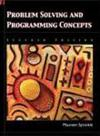Thông tin độc giả
Liên kết
Tài liệu được tìm trên toàn bộ hệ thống
327 tài liệu được tìm thấyKý hiệu xếp giá: 510.2855369D6741
THIS BOOK INCLUDES: GETTING ACQUAINTED; BASIC CONCEPTS; LISTS; TWO-DIMENSIONAL GRAPHICS; THREE -DIMENSIONAL GRAPHICS; EQUATIONS; ALGEBRA AND TRIGONOMETRY; DIFFERENTIAL CALCULUS; INTEGRAL CACULUS;...Ký hiệu xếp giá: 512.943Li-S
Contents Chapter 1 Vector in R" and C", spatial vectors Chapter 2 Algebra of matrices Chapter 3 Systems of linear equations Chapter 4 Vector spaces Chapter 5 Linear mappings Chapter 6 Linear mappings...Ký hiệu xếp giá: 510.285 536 9Do-E
Contents Chapter 1 GETTING ACQUAINTED Chapter 2 BASIC CONCEPTS Chapter 3 LISTS Chapter 4 TWO-DIMENSIONAL GRAPHICS Chapter 5 THREE -DIMENSIONAL GRAPHICS Chapter 6 EQUATIONS Chapter 7 ALGEBRA AND...Ký hiệu xếp giá: 332.801 51Zi-P
Contents Chapter 1 Exponents and logarithms Chapter 2 Progressions Chapter 3 Simple interest and simple discount Chapter 4 Compound interest and compound discount Chapter 5 Simple annuities Chapter 6...Ký hiệu xếp giá: 332.80151Z71
This book includes: Exponents and logarithms; Progressions; Simple interest and simple discount; Compound interest and compound discount; Simple annuities; General and other annuities; Amortization...Ký hiệu xếp giá: 658.304 5W556
This book includes: Bob's meltdown; A question of character; What a star-What a jerk; When your star performer can't manage; Do something-He's about to snap; When salaries aren't secret.Ký hiệu xếp giá: 519.5Mi-J
Contents Chapter 1 Introduction to probability and counting Chapter 2 Some probability laws Chapter 3 Discrete distributions Chapter 4 Continuous distributions Chapter 5 Joint distributions Chapter 6...Ký hiệu xếp giá: 519.5Mi-J
Contents Chapter 1 Introduction to probability and counting Chapter 2 Some probability laws Chapter 3 Discrete distributions Chapter 4 Continuous distributions Chapter 5 Joint distributions Chapter 6...Ký hiệu xếp giá: 005.133W431
Brief contents: Part I: objects and C++: arrays, pointers, and structures; objects and classes; templates; inheritance; design patterns; - Part II: Algorithms and building blocks: algorithm analysis;...Ký hiệu xếp giá: 005.1S767
Introduction to problem solving and programming; logic structures; data structures; database management systems; object-oriented programming; file processing; application software; supplementary...
Đang xem: 1152